# Explore hunderds of solved example topic wise.

## Java data types

### Data types

• In Java, every variable has some type.
• For example in the code snippet below x should be int and b should be boolean type variable.
`x = 10; b = true;`
• Similarly, In Java every expression also has some type.
• For example, In the following code snippet, a + b + c would be of int type because a, b and c are int type variables.

```int a, b, c, d;
a = 1; b = 1; c = 1;
d  = a + b + c;
```

### Java is strict type checked language

1. In Java, each type is strictly defined. For example, the byte ranges from -128 to 127, so value out of from this range is not acceptable by byte type.
2. Each assignment is strictly checked for type compatibility.
3. Due to the above two reasons, java is called strongly type-checked language.

### Primitive Data types in Java

Java has 8 primitive data types as listed below...

1. byte
2. short
3. int
4. long
5. float
6. double
7. char
8. boolean

The hierarchy of all 8 data types is shown by the following figure...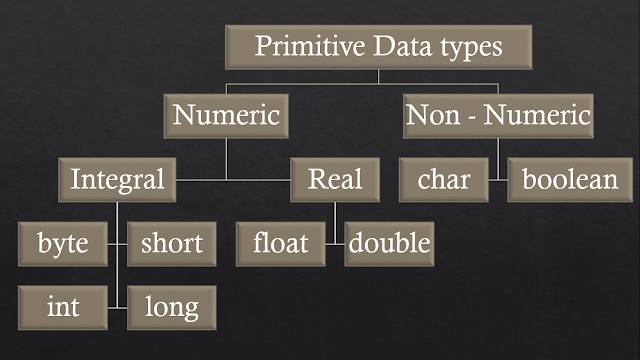### Singed unsigned data types

• Except for boolean and char, other primitive data types can be positive or negative.
• Data types that can have positive or negative values are called signed data types.
• Data type which can have only positive values are called unsigned data types, char and boolean are unsigned data types in Java.

The following code snippet shows the correct or incorrect values for different Data types.

```int a = 10; (✓)
int a = -10; (✓)
float x = -5.8; (✓)
char ch = -'a'; (✕)
boolean b = -true; (✕)
boolean b = true; (✓)
```

### byte data type in java

• Size: 1 byte (8 bits)
• Min Value: -128 (-27)
• Max Value: 127 (27 - 1)
• Range: -128 to 127 (-27 to 27 - 1)

### short data type in java

• Size: 2 byte (16 bits)
• Min Value: -32768 (-215)
• Max Value: 32767 (215 - 1)
• Range: -32768 to 32767 (-215 to 215 - 1)
The short data type is very rarely used data type.

### int data type in java

1. Size: 4 byte (32 bits)
2. Min Value: -2147483648 (-231)
3. Max Value: 2147483647 (231 - 1)
4. Range: -2147483648 to 2147483648 (-231 to 231 - 1)
int data type is mostly used data type in Java.

### long data type in java

1. Size: 8 byte (64 bits)
2. Min Value: -263
3. Max Value: 263 - 1
4. Range: -263 to 263 - 1

### float data type in java

If we want to store floating-point value, then we use a floating-point data type that can be either float or double data type.

1. Single Precision: 6-7 digit accuracy
2. Size: 4 byte (32 bits)
3. Min Value: -1.7e38 ( -1.7 * 1038 )
4. Max Value: 1.7e38 (1.7 * 1038)
5. Range: -1.7e38 to 1.7e38

### double data type in java

if you need store floating values with more precision (accuracy) then you should use double data type otherwise use float data type.

1. Double Precision: 14-15 digit accuracy
2. Size: 8 byte (64 bits)
3. Min Value: -3.4e308 ( -3.4 * 10308 )
4. Max Value: 3.4e308 (3.4 * 10308)
5. Range: -3.4e308 to 3.4e308

### boolean data type in java

1. Size: N/A (Depend upon JVM)
2. Range: N/A [Allowed values are false/true.]

#### Boolean Example-1:

```boolean b = 0;
System.out.println(b);
```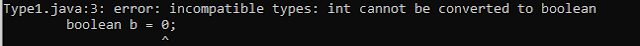#### Boolean Example-2:

```boolean b = "true";
System.out.println(b);
```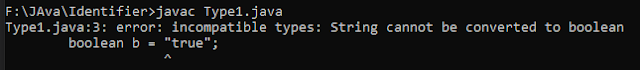#### Boolean Example-3:

```boolean b = True;
System.out.println(b);
```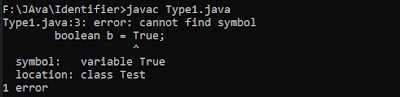#### Boolean Example-4:

```boolean b = true;
System.out.println(b); //true
```

#### Boolean Example-5:

```int a = 0;
if(a) {
System.out.println("Hello");
} else {
System.out.println("Bye");
}
```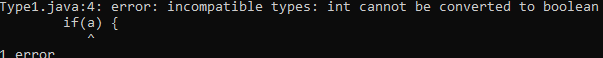### char data type in java

1. Size: 2 byte (16 bits)
2. Min Value: 0
3. Max Value: 65535 (216 - 1)
4. Range: 0 to 65535 (216 - 1)

### Java Data types summary

Data type Size Range Default Value
byte 1 byte -128 to 127 0
short 2 byte -32768 to 23767 0
int 4 byte -2147483648 to 2147483647 0
long 8 byte -231 to 231 - 1 0
float 4 byte -1.7 * 1038 to 1.7 * 1038 0.0f
double 8 byte -3.4 * 10308  to 3.4 to 10308 0.0
char 2 byte 0 to 65535 (0)\u0000
boolean N/A true / false false